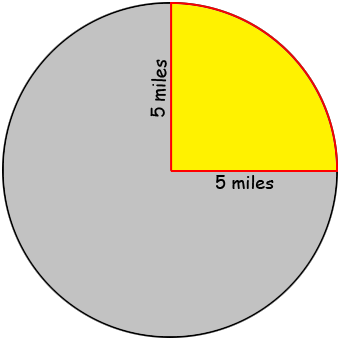SEARCH HOMEMath Central Quandaries & QueriesQuestion from Maisy, a student: This is the question that I got for a math worksheet. The radius of a quarter circle is 5 miles. What is the quarter circle's perimeter? Use 3.14 for pi. I don't remember how to solve this...Hi Maisy,

The one fact you need to know is that the circumference of a circle is $2 \pi \;r mbox{ miles}$ where $r$ is the radius in miles.In my diagram the quarter circle, coloured yellow, has a perimeter, coloured red, in three sections. Two of them are radii of the circle and the third is 1/4 of the circumference of the circle.

PennyMath Central is supported by the University of Regina and the Imperial Oil Foundation.